Hybrid Advection Scheme (Background)

March 1, 2014 by Joel E. Tohline

As is mentioned in the preface to our primary discussion of the Hybrid Advection Scheme, my early notes on this topic were included in my earliest version of this web-based H_Book. The relevant page can be accessed via this link; it is an html file whose linux time stamp is August 27, 2000. Here we re-present the content of these "year 2000" notes because the symbol fonts utilized throughout the original html page seem now not to be properly translated by some web browsers.

As my group discussed this proposed new algorithmic approach to advecting angular momentum over the first decade of the new millennium, it was often referred to as the "A* scheme," where,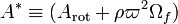$~A^* \equiv (A_\mathrm{rot} + \rho\varpi^2\Omega_f)$ was the inertial-frame angular momentum density as given by the sum of variables whose values were tracked in the rotating frame.

 |   Tiled Menu   |   Tables of Content   |  Banner Video   |  Tohline Home Page   |

ASIDE: Relevant to hydrocode development

Curvature Terms

Converting from a Lagrangian to an Eulerian time-derivative, Equation [I.A.5] becomes,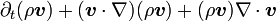$\partial_t(\rho\boldsymbol{v}) + (\boldsymbol{v}\cdot\nabla)(\rho \boldsymbol{v}) + (\rho \boldsymbol{v})\nabla\cdot \boldsymbol{v}$$~=~$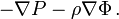$-\nabla P - \rho \nabla\Phi \, .$

Now, if you're not working in Cartesian coordinates, care must be taken when dealing with the second term on the left-hand-side of this equation because when the gradient operator acts on a vector quantity (in this case,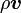$~\rho\boldsymbol{v}$), various curvature terms will arise reflecting the fact that, in general, the unit vectors of your curvilinear coordinate system point in different directions as the fluid moves to different locations in space. Quite generally, though, for the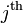$~j^\mathrm{th}$ component of the equation of motion we may isolate these curvature terms as follows: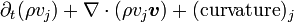$\partial_t(\rho v_j) + \nabla\cdot (\rho v_j \boldsymbol{v}) + (\mathrm{curvature})_j$$~=~$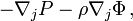$-\nabla_j P - \rho \nabla_j \Phi \, ,$

where,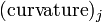$~(\mathrm{curvature})_j$$~=~$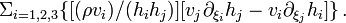$\Sigma_{i=1,2,3} \{ [ (\rho v_i)/(h_i h_j) ] [ v_j \partial_{\xi_i} h_j - v_i \partial_{\xi_j} h_i ] \} \, .$

So, for example, in cylindrical coordinates where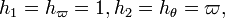$~h_1 = h_\varpi = 1, h_2 = h_\theta = \varpi,$ and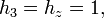$~h_3 = h_z = 1,$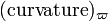$~(\mathrm{curvature})_\varpi$$~=~$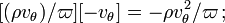$[ (\rho v_\theta)/\varpi ] [ -v_\theta ] = - \rho v_\theta^2/\varpi \, ;$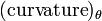$~(\mathrm{curvature})_\theta$$~=~$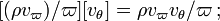$[ (\rho v_\varpi)/\varpi ] [ v_\theta ] = \rho v_\varpi v_\theta/\varpi \, ;$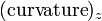$~(\mathrm{curvature})_z$$~=~$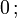$0 \, ;$

Thus, in cylindrical coordinates the three components of the equation of motion become,$\partial_t(\rho v_\varpi) + \nabla\cdot (\rho v_\varpi \boldsymbol{v})$$~=~$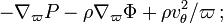$-\nabla_\varpi P - \rho \nabla_\varpi \Phi + \rho v_\theta^2/\varpi \, ;$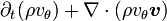$\partial_t(\rho v_\theta) + \nabla\cdot (\rho v_\theta \boldsymbol{v})$$~=~$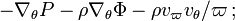$-\nabla_\theta P - \rho \nabla_\theta \Phi - \rho v_\varpi v_\theta/\varpi \, ;$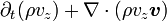$\partial_t(\rho v_z) + \nabla\cdot (\rho v_z \boldsymbol{v})$$~=~$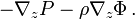$-\nabla_z P - \rho \nabla_z \Phi \, .$

Conservation of Angular Momentum

Now, the first and third of these expressions are indeed the ones we are utilizing in our hydrocode. but the middle one, expressing the time-rate-of-change of the azimuthal velocity, has been implemented in a different form, namely,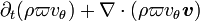$\partial_t(\rho \varpi v_\theta) + \nabla\cdot(\rho\varpi v_\theta \boldsymbol{v} )$$~=~$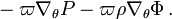$~ - \varpi \nabla_\theta P - \varpi \rho \nabla_\theta \Phi \, .$

The \$64,000 question is, "Are these equivalent expressions?" Well, let's play with the left-hand-side of this last expression.$\partial_t(\rho \varpi v_\theta) + \nabla\cdot(\rho\varpi v_\theta \boldsymbol{v} )$$~=~$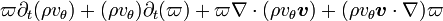$\varpi \partial_t(\rho v_\theta) + (\rho v_\theta) \partial_t(\varpi) + \varpi \nabla\cdot(\rho v_\theta \boldsymbol{v}) + (\rho v_\theta \boldsymbol{v}\cdot\nabla)\varpi$$~=~$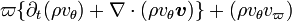$\varpi \{ \partial_t(\rho v_\theta) + \nabla\cdot(\rho v_\theta \boldsymbol{v}) \} + (\rho v_\theta v_\varpi)$

It is easy to see, therefore, that the two expressions are equivalent; but the latter one is used in preference to the former because it provides a direct statement of conservation of angular momentum. Specifically, when the external forces (due to gradients in the gravitational potential and pressure) balance, our selected "conservative" finite-difference scheme will guarantee that the physical quantity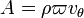$~A = \rho\varpi v_\theta$ is conserved globally to precisely the same degree of accuracy as mass is conserved.

In what follows, the governing PDEs will be expressed in terms of velocities that are measured in the context of a rotating reference frame. In our original html-formatted notes, these variables are differentiated from inertial-frame variables by color; specifically, rotating-frame variables are colored orange. Here we will abandon the color labeling and, instead, use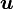$~\boldsymbol{u}$ to represent the velocity as measured in a frame rotating about the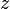$~z$-axis with angular frequency,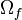$~\Omega_f$. That is,$~\boldsymbol{u}$ is related to the inertial-frame velocity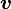$~\boldsymbol{v}$ through the expression,$~\boldsymbol{u}$$~=~$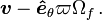$~\boldsymbol{v} - \boldsymbol{\hat{e}}_\theta \varpi\Omega_f \, .$

The So-called A* Scheme

Now, in a rotating frame of reference, this preferred form of the azimuthal component of the equation of motion takes the form,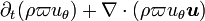$\partial_t(\rho \varpi u_\theta) + \nabla\cdot(\rho\varpi u_\theta \boldsymbol{u} )$$~=~$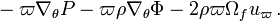$~ - \varpi \nabla_\theta P - \varpi \rho \nabla_\theta \Phi -2\rho\varpi \Omega_f u_\varpi \, .$

Notice that a new term has appeared due to the coriolis force. Traditionally, in numerical hydrodynamics, this new term has been treated explicitly as a source term. Hence, this component of the equation of motion no longer takes on a strictly conservative form, and the adopted "conservative" finite-difference is no longer a particularly useful tool to guarantee that the angular momentum is globally conserved even when the external forces due to pressure and gravity balance one another.

To derive a form of this equation that is a lot more suited to a "conservative" finite-difference implementation, note that,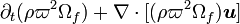$\partial_t (\rho \varpi^2 \Omega_f ) + \nabla\cdot [(\rho \varpi^2 \Omega_f)\boldsymbol{u}]$$~=~$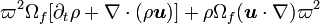$\varpi^2 \Omega_f [ \partial_t \rho + \nabla\cdot (\rho \boldsymbol{u})] + \rho \Omega_f (\boldsymbol{u}\cdot \nabla)\varpi^2$$~=~$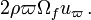$2\rho\varpi \Omega_f u_\varpi \, .$

Hence, in a rotating frame of reference, the azimuthal component of the equation of motion can be written as,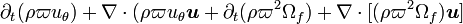$\partial_t (\rho\varpi u_\theta) + \nabla\cdot(\rho\varpi u_\theta \boldsymbol{u} + \partial_t (\rho\varpi^2 \Omega_f) + \nabla\cdot [ (\rho\varpi^2 \Omega_f) \boldsymbol{u} ]$$~=~$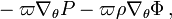$~ - \varpi \nabla_\theta P - \varpi \rho \nabla_\theta\Phi \, ,$

or,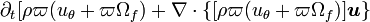$\partial_t [ \rho\varpi (u_\theta + \varpi\Omega_f) + \nabla\cdot \{ [ \rho\varpi (u_\theta + \varpi\Omega_f) ] \boldsymbol{u} \}$$~=~$$~ - \varpi \nabla_\theta P - \varpi \rho \nabla_\theta\Phi \, .$

When advancing the angular momentum density (i.e.,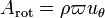$~A_\mathrm{rot} = \rho\varpi u_\theta$) forward in time using a finite-difference scheme, I recommend that the "sourcing" step only include the terms on the right-hand-side of these last expressions (i.e., only the gradients in the pressure and gravitational potential), and the "fluxing" step should include the following terms: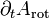$~\partial_t A_\mathrm{rot}$$~=~$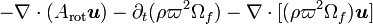$- \nabla\cdot (A_\mathrm{rot} \boldsymbol{u}) - \partial_t (\rho\varpi^2 \Omega_f) - \nabla\cdot [ ( \rho \varpi^2 \Omega_f) \boldsymbol{u} ]$$~=~$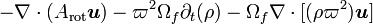$- \nabla\cdot (A_\mathrm{rot} \boldsymbol{u}) - \varpi^2 \Omega_f \partial_t (\rho ) - \Omega_f \nabla\cdot [(\rho\varpi^2) \boldsymbol{u} ]$$~=~$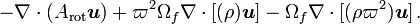$- \nabla\cdot (A_\mathrm{rot} \boldsymbol{u}) + \varpi^2 \Omega_f \nabla\cdot [(\rho) \boldsymbol{u}] - \Omega_f \nabla\cdot [(\rho\varpi^2) \boldsymbol{u} ] \, .$

This last expression is directly implementable using our standard fluxing scheme because all three terms have the form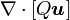$~\nabla\cdot[Q\boldsymbol{u}]$. (Note that the density that appears in the last two terms on the right-hand-side of this last expression must be taken from precisely the same point in time as the "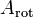$~A_\mathrm{rot}$" that appears in the first term on the right-hand-side.)

Whether you adopt precisely this final prescription or not, the primary point to keep in mind is that you want to advect the "intertial" [sic] angular momentum density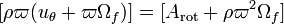$~[\rho\varpi(u_\theta + \varpi\Omega_f)] = [A_\mathrm{rot} + \rho\varpi^2 \Omega_f]$ using the "rotating-frame velocity$~\boldsymbol{u}$ at each grid cell face. Hence, you might prefer to use one slightly earlier relation to guide your design of the fluxing step. In the absence of "true" source terms (due to pressure and gravity), we have,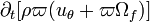$\partial_t [\rho \varpi (u_\theta + \varpi\Omega_f)]$$~=~$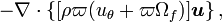$- \nabla\cdot\{[\rho\varpi ( u_\theta + \varpi\Omega_f)]\boldsymbol{u} \} \, ,$

which is the same as,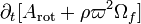$\partial_t [A_\mathrm{rot} + \rho\varpi^2\Omega_f ]$$~=~$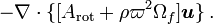$- \nabla\cdot\{[A_\mathrm{rot} + \rho\varpi^2\Omega_f ]\boldsymbol{u} \} \, .$

But this may also be written as,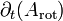$\partial_t (A_\mathrm{rot})$$~=~$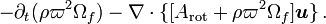$- \partial_t(\rho\varpi^2\Omega_f) - \nabla\cdot\{[A_\mathrm{rot} + \rho\varpi^2\Omega_f ]\boldsymbol{u} \} \, .$

So, you can carry out the calculation by first adding the quantity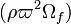$~(\rho\varpi^2 \Omega_f)$ to$~A_\mathrm{rot}$ to get the value of the angular momentum density in the inertial frame; advect this "inertial" quantity; then subtract from this result the change in the quantity$~(\rho\varpi^2 \Omega_f)$ as determined from the updated value of the density as derived via the continuity equation.

Related Discussions

 © 2014 - 2019 by Joel E. Tohline |   H_Book Home   |   YouTube   | Context: | PGE | SR | Appendices: | Equations | Variables | References | Binary Polytropes | Ramblings | Images | Images (2016 Layout) | ADS |